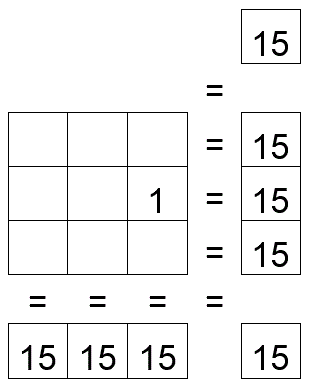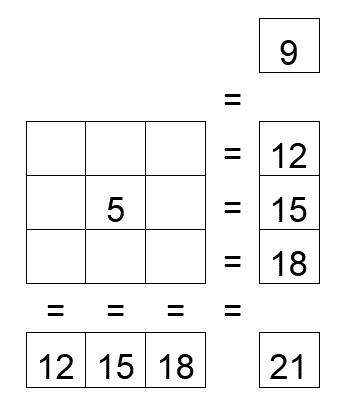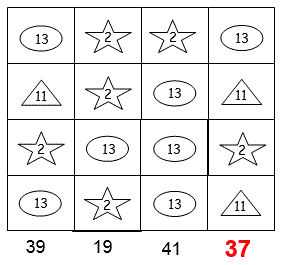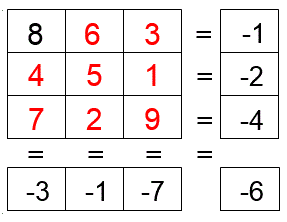Select Page

Each month, a new set of puzzles will be posted.  Come back next month for the solutions and a new set of puzzles, or subscribe to have them sent directly to you.

## MIND-Xpander puzzle

Twelve Dot Challenge – Connect the 12 dots with 5 straight lines without lifting your pen or pencil off the paper or going through any dot twice. There may be several solutions, depending on your starting point, with similar results.## Can-u-figure-it-out puzzle

How many squares, of any size, are there in the following diagram?## EQUATE-Sums puzzles

The missing numbers are between 1 & 9. Each number can be used only once. The numbers in each row add up to the totals on the right, and the columns adds up to the totals along the bottom. The sum of the two diagonals are also given. Solve the puzzle by finding all the missing numbers that satisfy all the sums as shown.## Feedback

There are more than one way of doing these puzzles and may well be more than one answer.  Please let me and others know what alternatives you find by commenting below.  We also welcome general comments on the subject and any feedback you'd like to give.

If you have a question that needs a response from me or you would like to contact me privately, please use the contact form.

## Get more puzzles!

If you've enjoyed doing the puzzles, consider ordering the books;

• Book One - 150+ of the best puzzles
• Book Two - 200+ with new originals and more of your favourites

Both in a handy pocket sized format.   Click here for full details.

## MIND-Xpander numeric puzzles

1. The dimensions of one box are all two-thirds of the dimensions of another. If the volume of the smaller is 64 cubic centimetres, what is the volume of the larger box?   Solution: The dimensions of the smaller box are 4 x 4 x 4 = 64. The dimensions of the larger box are 3/2 x 4 = 6. Therefore, volume of the larger box is 6 x 6 x 6 = 216 cu. cm. or (3/2 x 3/2 x 3/2) x 64 = (27/8) x 64 = 216 cu. cm.
2. Arrange the digits 1, 2, 3, 4 and 5, using only plus & minus signs (addition & subtraction), so that they will total 111. Using the same instructions, arrange the digits for totals of 222, and 333. (Note: There may be more than one solution.)   Solutions: 135 – 24 = 111,  214 + 3 + 5 = 222,  345 – 12 = 333

## Equate-Sums puzzle

Can you work out what each of the four symbols are worth? The totals of the three columns are given.  Can you also work out the missing total?

Solution:## Equate-TakeAway puzzle

The missing numbers are between 1 & 9.  The three numbers in top columns, when subtracted by the lower two numbers, equals the three totals along the bottom, and the three numbers in the left rows, when subtracted by the right two numbers, equals the three totals at the far right.  The top left number, when subtracted by the lower two numbers on its diagonal, equals the total in the bottom, far right box.  With one number provided, solve the puzzle by finding all missing numbers that satisfy all of the results as shown